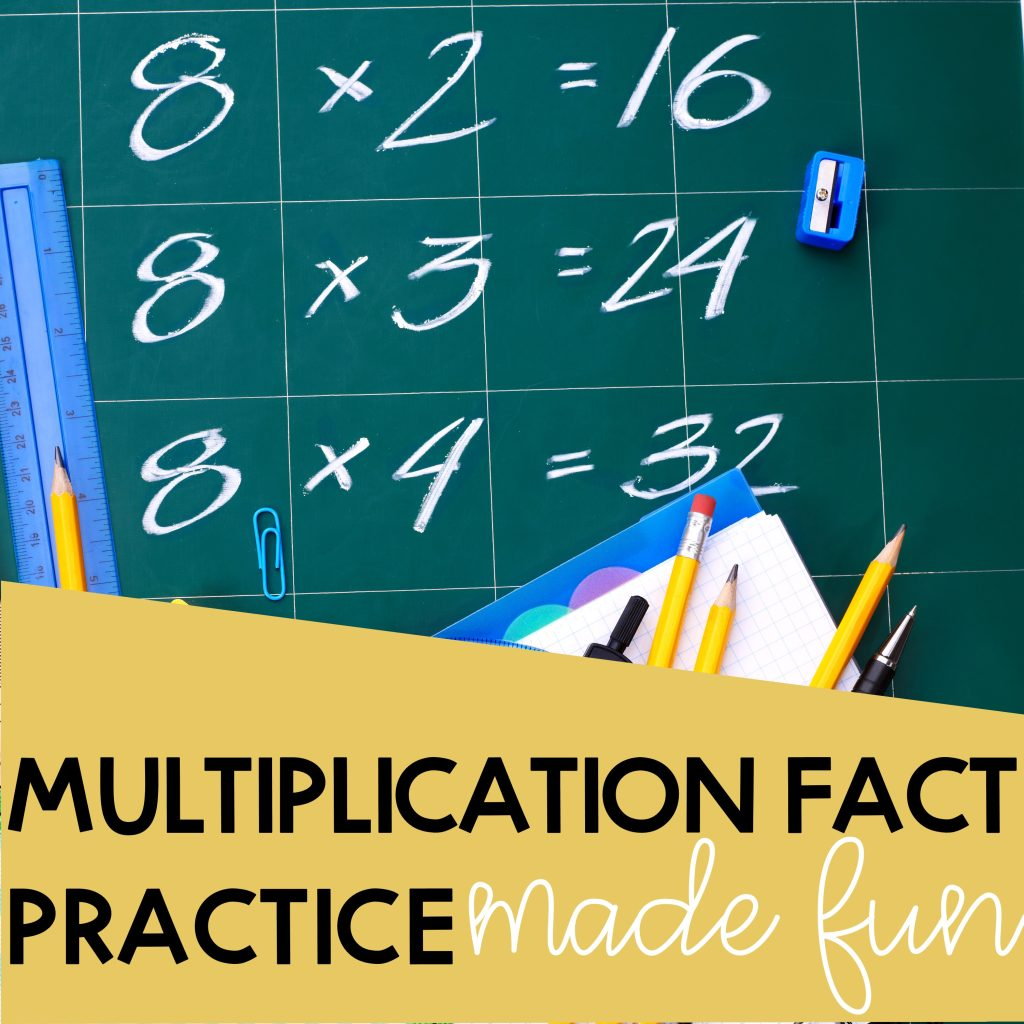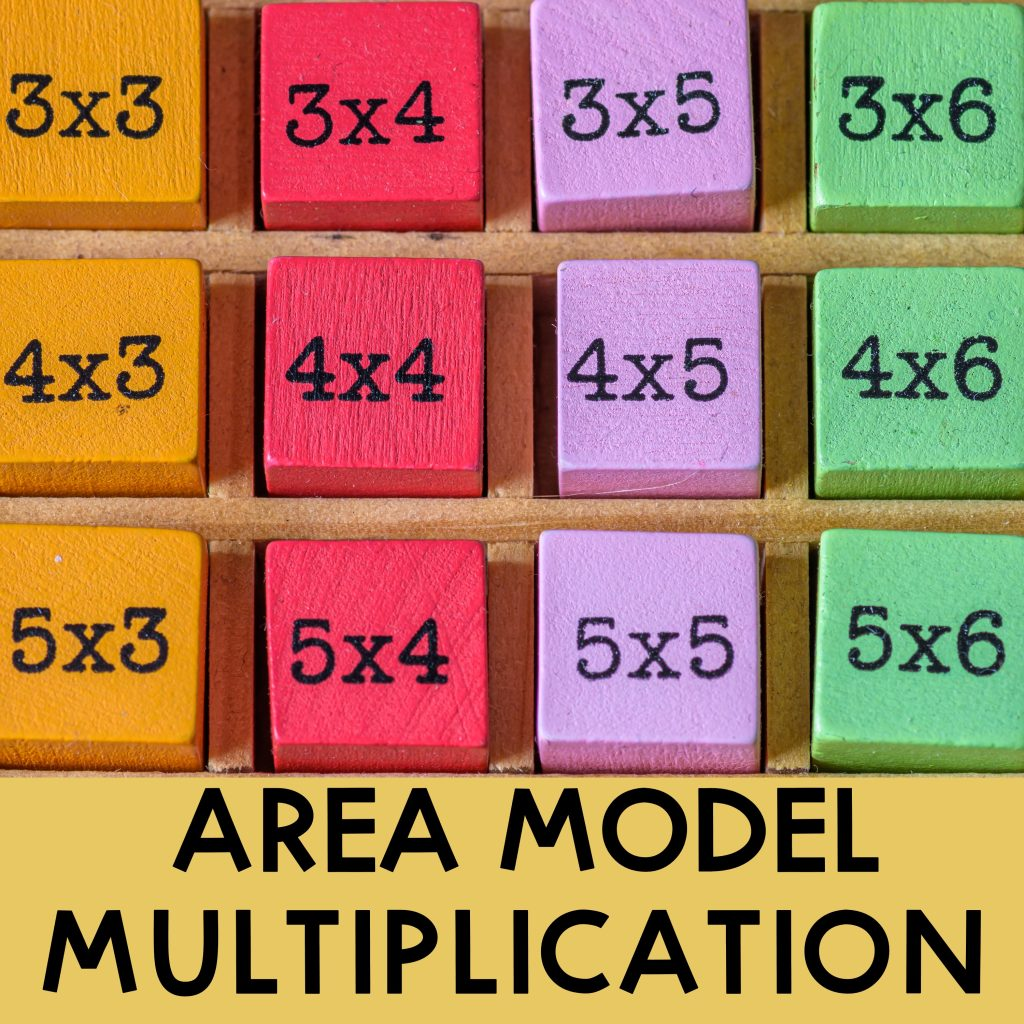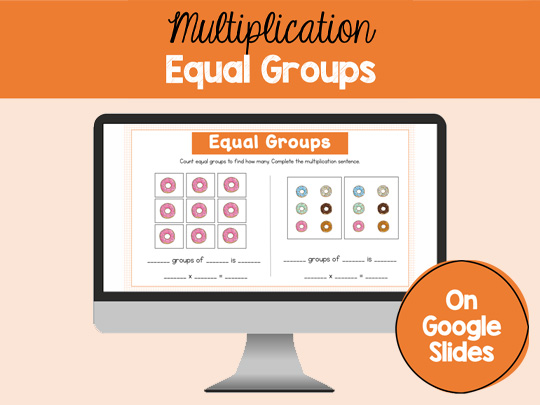# multiplication## Multiplication Facts Practice Made Fun

If you need multiplication fact practice, flashcards and timed tests are not your only option. Multiplication facts are really important in elementary math. Knowing multiplication facts by heart will give elementary students the confidence to tackle more complex problems in the future. Not only that, but they are more likely to use these facts in …## Area Model Multiplication: Guide and Examples

Area model multiplication is a great strategy when learning multi-digit multiplication. They help break down the numbers into easy-to-multiply digits and make multiplying friendly. In this article, you will learn how to use an area model to multiply 2-, 3-, and 4-digit numbers by 1-digit numbers and 2-digit numbers by 2-digit numbers. Area Model Multiplication: …## Understanding Multiplication: Equal Groups

Learning multiplication is not as easy as just memorizing the facts. That may help later on, but without understanding the concept of multiplication, it’s really nothing. Start teaching multiplication using equal groups to have a concrete introduction to multiplication. One of the best things to do is to relate multiplication with things  that they already know. …